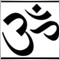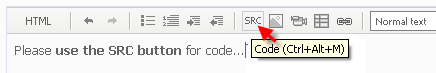# why the value of them is zero53

Dear expert:

I use history data of auto trade system to test the function,the value of RSV is zero,Please tell me why?

while(i>=0)
{
MaxHigh=High[iHighest(NULL,0,MODE_HIGH,nPeriod,i)];
MinLow=Low[iLowest(NULL,0,MODE_LOW,nPeriod,i)];
if (MaxHigh-MinLow != 0) RSV[i]=(Close[i]-MinLow)/(MaxHigh-MinLow)*100;
else RSV[i] = 50;
i--;
}

thanks!53

If anyone can give me answer?

thanks!5299

What is RSV? Use print to check the value of i.53

RSV is a buffer, if I set a element of it to a value, for example,

RSV = 50,I print it, it displays 0, why?17271

sniff:

RSV is a buffer, if I set a element of it to a value, for example,

RSV = 50,I print it, it displays 0, why?

How did you declare the buffer/array ? is it a Buffer or an Array ? is this in an Indicator or an EA ?53

it is a global variable

double RSV[];17271

sniff:

it is a global variable

double RSV[];

OK, if it's not a Indicator buffer you have to define how many elements are in the Array, otherwise it has 0 elements and then all it's values . . . all 0 of them, are 0.

You need to do this . . .

`double RSV;  <------ adjust to the number you need or use ArrayResize()`53

Do you know how to get the value of array element?

thanks!17271

sniff:

Do you know how to get the value of array element?

thanks!

Yes . . . arrayname[arrayelementnumber], note, first element number is 022318

sniff:

RSV is a buffer, if I set a element of it to a value, for example,

RSV = 50,I print it, it displays 0, why?

If it displays zero, how do you know it's 50?53

thank your help, the value of RSV is correct.there is another question,please see the code.

double percentK;

double RSV;

limit=Bars-counted_bars;
if(limit > 512) limit = 512;
//---- signal line is simple movimg average
for(i=0; i<limit; i++)
{
percentK[i]=iMAOnArray(RSV,Bars,M1,0,MODE_SMA,i);

}

the percentK[i] value is -0,why?